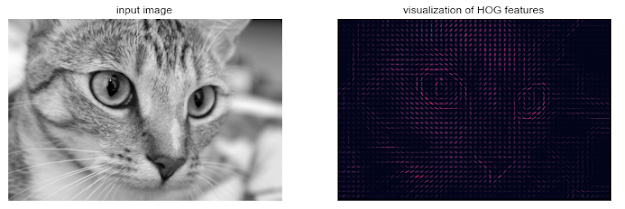## 2010年10月4日 星期一

### [ 資料結構 小學堂 ] 佇列 : 認識佇列1. 具有先進先出 (FIFO) 的特性.
2. 擁有兩種基本動作加入與刪除, 而且使用 front 與 rear 兩個指標來分別指向佇列的前端與尾端.

* CREATE : 建立空佇列
* FRONT : 傳回佇列前端的值
* DELETE : 刪除佇列前端的值, 並傳回新佇列.
* EMPTY : 若佇列為空集合, 則為真, 否則為偽.

* JQueue.h 代碼 :

1. #include
2. #include
3.
4. using namespace std;
5. #define MAX_QUEUE_SIZE 20 // 定義佇列的大小
6.
7. class JQueue{
8. protected:
9.     int rear;
10.     int _queue[MAX_QUEUE_SIZE];
11. public:
12.     JQueue(){
13.         rear = -1;
14.     }
15.     bool add(int val);  // 添加新資料到佇列
16.     bool isEmpty();  //判斷佇列是否為空
17.     bool isFull();  // 判斷佇列是否已滿
18.     bool get(int *val); // 取回佇列的值
19.     int length(); // 目前佇列的資料數量.
20. };
* JQueue.cpp 代碼 :

1. #include "JQueue.h"
2.
4.     if(!this->isFull()) {
5.         rear++;
6.         this->_queue[rear] = val;
7.         return true;
8.     }
9.     cout << "[佇列已滿]" << endl;
10.     return false;
11. }
12.
13. bool JQueue::isFull() {
14.     if((rear+1)==MAX_QUEUE_SIZE) {
15.         return true;
16.     } else {
17.         return false;
18.     }
19. }
20.
21. bool JQueue::isEmpty() {
22.     if(rear>=0) {
23.         return false;
24.     } else {
25.         return true;
26.     }
27. }
28.
29. bool JQueue::get(int *val) {
30.     if(!this->isEmpty()) {
31.         *val = this->_queue;
32.         for(int i=0; i
33.             this->_queue[i] = this->_queue[i+1];
34.         }
35.         rear--;
36.         return true;
37.     } else {
38.         cout << "[佇列為空!]" << endl;
39.         *val = -1;
40.         return false;
41.     }
42. }
43.
44. int JQueue::length(){
45.     return rear+1;
46. }
* 呼叫演算法代碼 :

1. void ch05_01(bool b) {
2.     if(b) {
3.         char ch = 'S';
4.         int val;
5.         int gval=-1;
6.         JQueue queue;
7.         while(ch != 'E') {
8.             cout << "[A]存入一個數值, [G] 取出一個數值, [E] 結束, [L] 目前佇列長度 :";
9.             cin >> ch;
10.             switch(ch) {
11.                 case 'A':
12.                     cout << "請輸入數值: ";
13.                     cin >> val;
15.                     break;
16.                 case 'G':
17.                     queue.get(&gval);
18.                     if(gval>=0) {
19.                         cout << "取出數值為: " << gval << endl;
20.                     }
21.                     break;
22.                 case 'E':
23.                     cout << "[離開程式]" << endl;
24.                     break;
25.                 case 'L':
26.                     cout << "目前佇列資料數目為: " << queue.length() << endl;
27.                     break;
28.                 default:
29.                     cout << "不合法字元! (" << ch << ")"  << endl;
30.                     break;
31.             }
32.         }
33.     }
34. }

[A]存入一個數值, [G] 取出一個數值, [E] 結束, [L] 目前佇列長度 :A <添加新資料>

[A]存入一個數值, [G] 取出一個數值, [E] 結束, [L] 目前佇列長度 :A <添加新資料>

[A]存入一個數值, [G] 取出一個數值, [E] 結束, [L] 目前佇列長度 :L <檢查目前佇列資料數目>

[A]存入一個數值, [G] 取出一個數值, [E] 結束, [L] 目前佇列長度 :G <取回佇列資料>

[A]存入一個數值, [G] 取出一個數值, [E] 結束, [L] 目前佇列長度 :G

[A]存入一個數值, [G] 取出一個數值, [E] 結束, [L] 目前佇列長度 :G
[佇列為空!]
[A]存入一個數值, [G] 取出一個數值, [E] 結束, [L] 目前佇列長度 :E <離開程式>
[離開程式]

* [ 資料結構 小學堂 ] 堆疊 : 認識堆疊

### [Py DS] Ch5 - Machine Learning (Part14)

Application: A Face Detection Pipeline This chapter has explored a number of the central concepts and algorithms of machine learning. But mo...